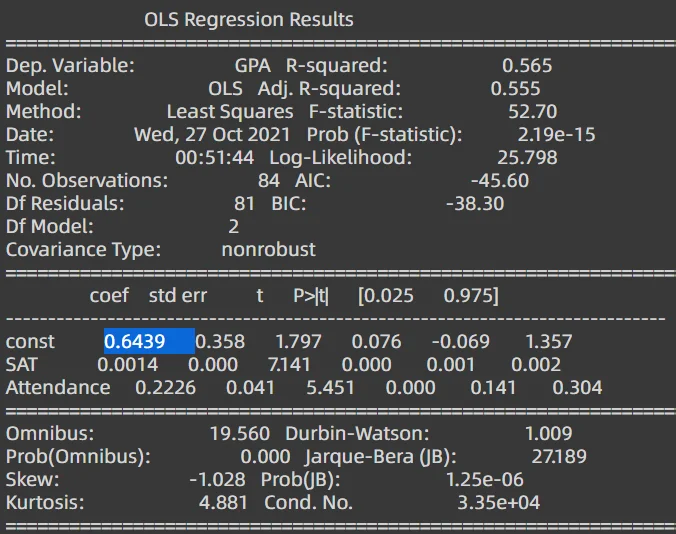27 Oct 2021

Posted on:

27 Oct 2021

1

# Resolved:Question about the yhat_yes and yhat_no equation

How do you get the yhat_yes and yhat_no equation? Thank you so much! I could not find it in the summary table

Instructor
Posted on:

27 Oct 2021

0

Hey Jackie,

Thank you for the question!

Once we include the dummy variable into our regression model, the equation becomes:

GPA = 0.6439 + 0.0014 * SAT + 0.2226 * Dummy

What the coefficients' table can give you are the bias (called `const` in the table) as well as the coefficients in front of the SAT variable and the Dummy variable (called `Attendance` in the table). These values are 0.6439, 0.0014 and 0.2226, respectively. The table doesn't give you the values for `yhat_no` and `yhat_yes`. What we need to do to obtain them is the following.

The Dummy variable can take 2 different values:

1. For people who did not attend, Dummy = 0. Therefore, the equation above becomes simply:
GPA = 0.6439 + 0.0014 * SAT

This equation is defined as `yhat_no` in the code:

`yhat_no = 0.6439 + 0.0014 * data['SAT']`

2. For people who did attend classes, Dummy = 1. Therefore, the first equation becomes:
GPA = 0.6439 + 0.0014 * SAT + 0.2226 * Dummy = 0.6439 + 0.0014 * SAT + 0.2226 * 1 = 0.6439 + 0.2226 + 0.0014 * SAT = 0.8665 + 0.0014 * SAT

This equation is defined as `yhat_yes` in the code:

`yhat_yes = 0.8665 + 0.0014 * data['SAT']`

We now plot `data['SAT']` on the x-axis. On the y-axis, we plot both `yhat_no` (the line that appears lower) and `yhat_yes` (the line that appears higher).

Hope this helps! Keep up the good work!

Kind regards,
365 Hristina